# Intersecting tangent problem

• Bounceback
In summary: If ##q(x) = x^4 + cx^3 + 12 x^2 - 5x + 2 - (ax+b)##, the tangency requirement means that ##q(x)## has a zero of multiplicity 2 at some point. That means that there can only be TWO other zeros of ##q(x)##, not three. In other words, there are only three distinct points of intersection, not four.If ##q(x)## is our original polynomial, we just interested for the values of x for which ##p(x)=ax+b## so we want to find the values of c (and possibly the values of a,b if it

#### Bounceback

For what values of c is there a straight line that intersects the curve in four distinct places?
$x^4+c*x^3+12x^2-5x+2$
This is what the equation looks like where c=7 (red) and c=6 (blue), to help get an idea.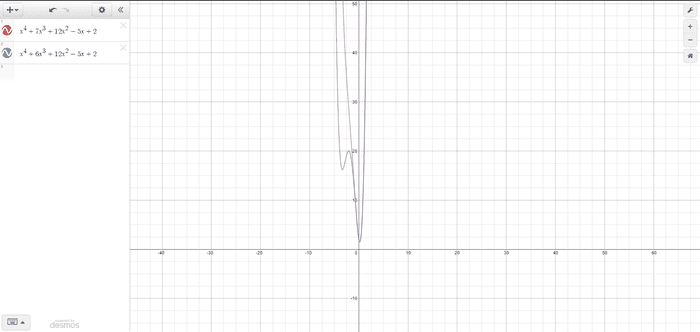There can always be a straight line intersecting four points while the bottom two 'humps' have a common tangent line. I'm looking to find the value of c where that left hump disappears, which can be found when there are no two points that share a common tangent line.

Starting now, finding the general tangent equation:
$y=(4a^3+3*c*a^2+12a-5)*x+b$ Note, a is the x value that the tangent is built from

Since both the tangent and the equation share one known point (where x=a), we can find b
$x^4+c*x^3+12x^2-5x+2=(4a^3+3*c*a^2+12a-5)*x+b$
$a^4+c*a^3+12a^2-5a+2=4a^4+3*c*a^3+12a^2-5a+b$
$b=-3a^4-2*c*a^3-12a^2+2$

This gives us the general equation of:
$y=(4a^3+3*c*a^2+24a-5)x-3a^4-2*c*a^3-12a^2+2$

On the two points that share a common tangent line, the height of both the tangent line and the original function will be the same. This allows us to solve for x:
$x^4+c*x^3+12x^2-5x+2=(4a^3+3*c*a^2+24a-5)x-3a^4-2*c*a^3-12a^2+2$
$x^4+c*x^3+12x^2-5x-(4a^3+3*c*a^2+24a-5)x+3a^4+2*c*a^3+12a^2=0$

The four roots of this equations are:
x and a are both on the bottom 'hump'
x and a are both on the top 'hump'
x is on the bottom 'hump' and a is on the top
x is on the top 'hump' and a is on the bottom

For the first two options, x=a. So the last equation can be divided by x=a twice.
$\frac{x^4+c*x^3+12x^2-5x-(4a^3+3*c*a^2+24a-5)x+3a^4+2*c*a^3+12a^2}{(x-a)^2}=0$
$x^2+(2a+c)x+3a^2+2*c*a+12=0$

Solving for x yields:
$x=\frac{-2a-c\pm SQRT(c^2-4*c*a-8a^2-48)}{2}$

This formula finds x (the second point that shares a common tangent line) in terms of the first point that shares the common tangent line. The square root allows us to find where a common tangent line is shared, where the stuff inside the square root is less than 0, no real value for x exists, meaning there is no second point that shares a common tangent line.

$c^2-4*c*a-8a^2-48>0$
$c>\frac{4a\pm SQRT(16a^2+32a^2+192)}{2}$
$c>2a\pm 2*SQRT(3a^2+12)$

From here, I'm stuck
I'm looking for a full answer (doesn't have to use the same method)

The equation of curve is y = x⁴ + cx³ +12x² -5x +2. Let the line be y = mx +d. The x coordinate of the points of intersection will satisfy the equation
mx + d = x⁴ + cx³ +12x² -5x +2 or
x⁴ + cx³ +12x² -(5+m)x + (2-d) = 0. This equation must have four distinct real roots. If α, β, γ and δ are the real distinct roots then we have
α + β + γ + δ = - c ----------------- (1)
αβ + βγ + γδ + δα = 12 ---------- (2)
αβγ + βγδ + γδα + δαβ = 5+m -----(3) and
αβγδ = 2-d ------------------------ (4)
There are 3 unknowns and 4 equations and we need to find only the value of c.

•Delta2
Sorry I am confused about the exact statement of the problem, we want to find a line that is tangent at some point and it intersects the given polynomial at 3 more points, OR we want to find any line that intersects it at 4 points?

if it is the latter then if ##p(x)## is our original polynomial, we just interested for the values of x for which ##p(x)=ax+b## so we want to find the values of c (and possibly the values of a,b if it depends on those too) for which the polynomial ##q(x)=p(x)-ax-b## has 4 distinct real roots.

Bounceback said:
For what values of c is there a straight line that intersects the curve in four distinct places?
$x^4+c*x^3+12x^2-5x+2$
This is what the equation looks like where c=7 (red) and c=6 (blue), to help get an idea.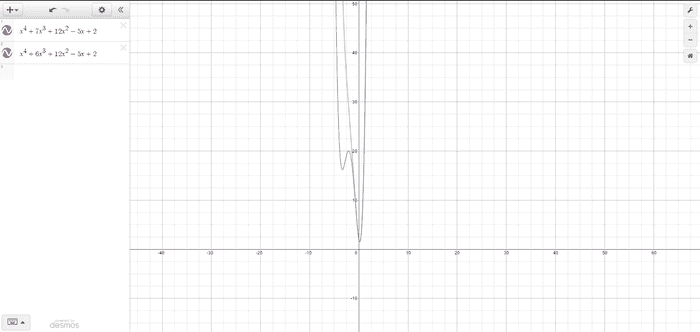There can always be a straight line intersecting four points while the bottom two 'humps' have a common tangent line. I'm looking to find the value of c where that left hump disappears, which can be found when there are no two points that share a common tangent line.

Starting now, finding the general tangent equation:
$y=(4a^3+3*c*a^2+12a-5)*x+b$ Note, a is the x value that the tangent is built from

Since both the tangent and the equation share one known point (where x=a), we can find b
$x^4+c*x^3+12x^2-5x+2=(4a^3+3*c*a^2+12a-5)*x+b$
$a^4+c*a^3+12a^2-5a+2=4a^4+3*c*a^3+12a^2-5a+b$
$b=-3a^4-2*c*a^3-12a^2+2$

This gives us the general equation of:
$y=(4a^3+3*c*a^2+24a-5)x-3a^4-2*c*a^3-12a^2+2$

On the two points that share a common tangent line, the height of both the tangent line and the original function will be the same. This allows us to solve for x:
$x^4+c*x^3+12x^2-5x+2=(4a^3+3*c*a^2+24a-5)x-3a^4-2*c*a^3-12a^2+2$
$x^4+c*x^3+12x^2-5x-(4a^3+3*c*a^2+24a-5)x+3a^4+2*c*a^3+12a^2=0$

The four roots of this equations are:
x and a are both on the bottom 'hump'
x and a are both on the top 'hump'
x is on the bottom 'hump' and a is on the top
x is on the top 'hump' and a is on the bottom

For the first two options, x=a. So the last equation can be divided by x=a twice.
$\frac{x^4+c*x^3+12x^2-5x-(4a^3+3*c*a^2+24a-5)x+3a^4+2*c*a^3+12a^2}{(x-a)^2}=0$
$x^2+(2a+c)x+3a^2+2*c*a+12=0$

Solving for x yields:
$x=\frac{-2a-c\pm SQRT(c^2-4*c*a-8a^2-48)}{2}$

This formula finds x (the second point that shares a common tangent line) in terms of the first point that shares the common tangent line. The square root allows us to find where a common tangent line is shared, where the stuff inside the square root is less than 0, no real value for x exists, meaning there is no second point that shares a common tangent line.

$c^2-4*c*a-8a^2-48>0$
$c>\frac{4a\pm SQRT(16a^2+32a^2+192)}{2}$
$c>2a\pm 2*SQRT(3a^2+12)$

From here, I'm stuck
I'm looking for a full answer (doesn't have to use the same method)
The title of the thread mentions a tangent. The statement of the problem does not mention a tangent.

Please give the complete statement of the problem in the body of the thread, no matter what the thread title states.

Bounceback said:
For what values of c is there a straight line that intersects the curve in four distinct places?
$x^4+c*x^3+12x^2-5x+2$
This is what the equation looks like where c=7 (red) and c=6 (blue), to help get an idea.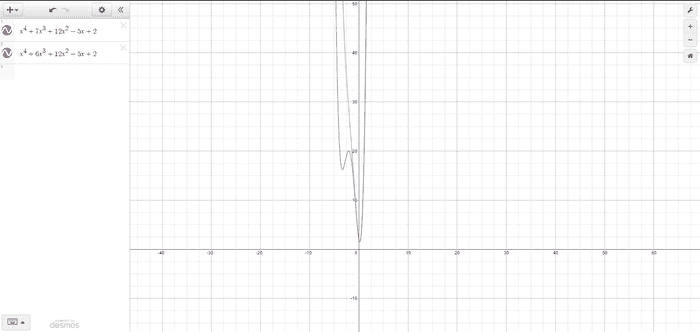There can always be a straight line intersecting four points while the bottom two 'humps' have a common tangent line. I'm looking to find the value of c where that left hump disappears, which can be found when there are no two points that share a common tangent line.

Starting now, finding the general tangent equation:
$y=(4a^3+3*c*a^2+12a-5)*x+b$ Note, a is the x value that the tangent is built from

Since both the tangent and the equation share one known point (where x=a), we can find b
$x^4+c*x^3+12x^2-5x+2=(4a^3+3*c*a^2+12a-5)*x+b$
$a^4+c*a^3+12a^2-5a+2=4a^4+3*c*a^3+12a^2-5a+b$
$b=-3a^4-2*c*a^3-12a^2+2$

This gives us the general equation of:
$y=(4a^3+3*c*a^2+24a-5)x-3a^4-2*c*a^3-12a^2+2$

On the two points that share a common tangent line, the height of both the tangent line and the original function will be the same. This allows us to solve for x:
$x^4+c*x^3+12x^2-5x+2=(4a^3+3*c*a^2+24a-5)x-3a^4-2*c*a^3-12a^2+2$
$x^4+c*x^3+12x^2-5x-(4a^3+3*c*a^2+24a-5)x+3a^4+2*c*a^3+12a^2=0$

The four roots of this equations are:
x and a are both on the bottom 'hump'
x and a are both on the top 'hump'
x is on the bottom 'hump' and a is on the top
x is on the top 'hump' and a is on the bottom

For the first two options, x=a. So the last equation can be divided by x=a twice.
$\frac{x^4+c*x^3+12x^2-5x-(4a^3+3*c*a^2+24a-5)x+3a^4+2*c*a^3+12a^2}{(x-a)^2}=0$
$x^2+(2a+c)x+3a^2+2*c*a+12=0$

Solving for x yields:
$x=\frac{-2a-c\pm SQRT(c^2-4*c*a-8a^2-48)}{2}$

This formula finds x (the second point that shares a common tangent line) in terms of the first point that shares the common tangent line. The square root allows us to find where a common tangent line is shared, where the stuff inside the square root is less than 0, no real value for x exists, meaning there is no second point that shares a common tangent line.

$c^2-4*c*a-8a^2-48>0$
$c>\frac{4a\pm SQRT(16a^2+32a^2+192)}{2}$
$c>2a\pm 2*SQRT(3a^2+12)$

From here, I'm stuck
I'm looking for a full answer (doesn't have to use the same method)

If ##q(x) = x^4 + cx^3 + 12 x^2 - 5x + 2 - (ax+b)##, the tangency requirement means that ##q(x)## has a zero of multiplicity 2 at some point. That means that there can only be TWO other zeros of ##q(x)##, not three. In other words, there are only three distinct points of intersection, not four.

You can also see this geometrically: imagine drawing graphs of the equations ##y = x⁴ + cx³ +12x² -5x +2## and ##y = ax + b##. These generally intersect at 4 distinct points. Now imagine sliding the line ##y = ax + b## up or down until you make two of the intersection points (on opposite sides of a peak or valley) coalesce into one. The line will then be tangent at one point and will intersect the curve at two other points. Essentially, two of the four points have blended into one point, leaving two others.

Last edited:
To clarify, this question was obtained from a list of problems involving tangents. The straight line does not need to lie on a tangent, that was my way of working the problem out. Also, my understanding of the question is that it is asking for a range for C, not one single value.

To clarify why I'm trying to find a tangent that is shared by two points: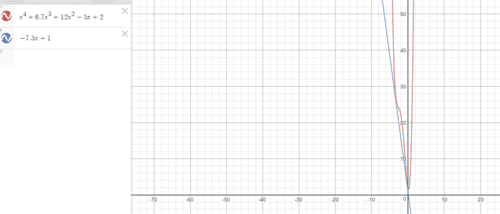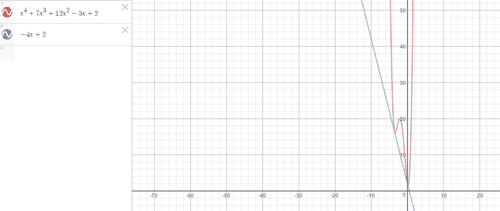As the C value decreases, the higher 'hump' gets higher and begins disappearing. I'm assuming that that top hump eventually disappears. My solution attempt above is trying to find the moment the hump disappears, or rather, the moment that two points cannot share a common tangent line.
Below for example, there looks like no top 'hump' and so looks like there's no two points that share the same tangent.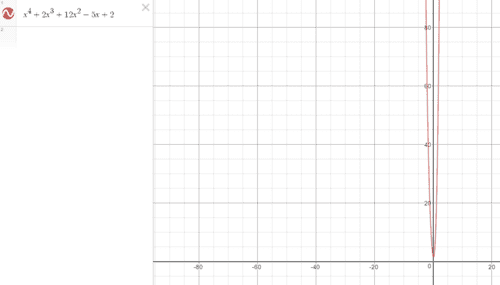Let'sthink said:
The equation of curve is y = x⁴ + cx³ +12x² -5x +2. Let the line be y = mx +d. The x coordinate of the points of intersection will satisfy the equation
mx + d = x⁴ + cx³ +12x² -5x +2 or
x⁴ + cx³ +12x² -(5+m)x + (2-d) = 0. This equation must have four distinct real roots. If α, β, γ and δ are the real distinct roots then we have
α + β + γ + δ = - c ----------------- (1)
αβ + βγ + γδ + δα = 12 ---------- (2)
αβγ + βγδ + γδα + δαβ = 5+m -----(3) and
αβγδ = 2-d ------------------------ (4)
There are 3 unknowns and 4 equations and we need to find only the value of c.
I don't have much experience in this area, there looks like 7 unknowns however ($\alpha, \beta, \gamma, \delta$, c, m and d). Could you explain how you would go on from here?

Last edited: